# Working with lists in Python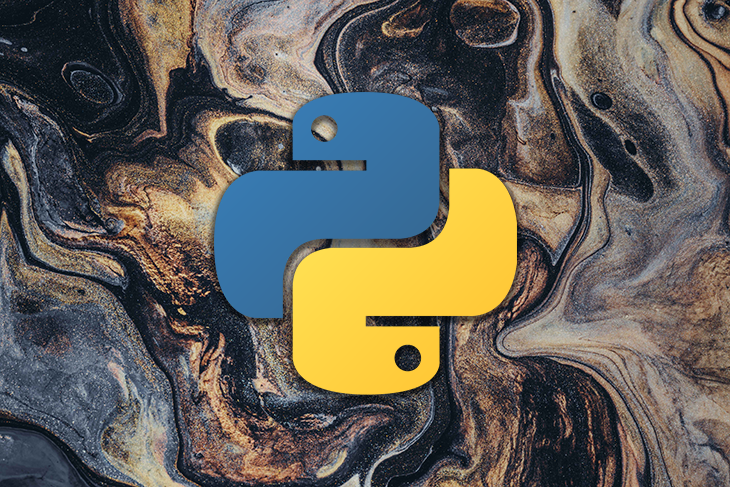Python is a popular general-purpose language among web developers, data scientists, machine learning engineers, and system administrators. Nowadays, we can also use Python to build cross-platform mobile applications, with Kivy. Python is becoming more popular every day due to its simplicity, beginner friendliness, and rich plugin ecosystem.

We can create variables to store single data records in Python like any other dynamically-typed language. In our Python programs, we often have to save a sequence of data records in one variable. For those scenarios, we can use the Python list data structure, which allows you to create and manipulate multiple items with one variable.

In this tutorial, I will explain everything you need to know about Python lists with practical examples. Also, we’ll discuss some advanced topics related to lists, such as multi-dimension lists, mapping, and filtering.

## Prerequisites

You’ll need to install the Python 3 interpreter to get started. In most Linux distributions, Python 3 is pre-installed.

In this tutorial, I will be using the `python3` command to execute Python scripts because I am demonstrating on Linux.

## List syntax and principles

A Python list is a comma-separated list of elements surrounded by two square brackets. You can add any element type to a list. Add the following code to a new file named `main.py` to create a simple list.

```languages = ['Python', 'Go', 'Dart']
print(type(languages))
print(languages)
print(len(languages))
```

Now, run the above code with your Python interpreter’s binary name.

```python3 main.py
```

The above code creates a new list with three string elements. Also, it prints the `languages` variable’s type, contents, and item count, as shown below.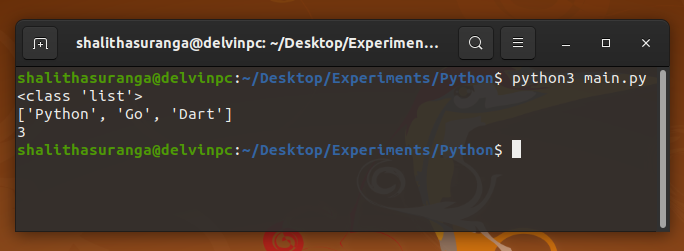As you can see, the `len` function returns the current item count of the list.

You can access each element by providing the index within two square brackets. The list indexing starts from `0`, like generic arrays in computer science. For example, the following code prints the second element’s value:

```languages = ['Python', 'Go', 'Dart']
print('The second element: ' + languages) # Go
```

In Python, you can enter minus values for indices. `-1` refers to `len(list) - 1` (last element), `-2` refers to `len(list) - 2` (element before the last element), and so on. For example, if you change the second code line as below, you will get “Dart” as the output.

```print('The last element: ' + languages[-1]) # Dart
```

You can also check the existence of item with the `in` keyword.

```languages = ['Python', 'Go', 'Dart']
if 'Python' in languages:
print('I <3 Python')
```

## Creating and initializing new lists

We’ve initialized the previous list with pre-defined values. There are some other ways to initialize lists, too.

### Initializing lists without values

If you don’t know the list’s content upfront, you can create an empty list and populate it later. For example, the following code creates the previous `languages` list by creating an empty list at the beginning.

```languages = []
languages.append('Python')
languages.append('Go')
languages.append('Dart')
print(languages) # ['Python', 'Go', 'Dart']
```

Here we used the `append` method to add a new element to the list.

### Initializing lists with generated values

We often need to generate instant lists. For example, sometimes we have to create integer lists, such as `1` to `10`, `1` to `100`, `2` to `50`, etc. Instead of writing them all out, we can use the inbuilt `range` function to create these simple number sequences. The following code creates a list that has integers between `1` and `10`.

```A = list(range(1, 10))
print(A) # [1, 2, 3, 4, 5, 6, 7, 8, 9]
```

In the above code, the `range` function returns a range object, so we have to use the `list` function to get a new list from the range object. We can use the `range` function’s third parameter to add gaps or steps between numbers. For example, the following code generates a list of even numbers between `0` and `10`.

```A = list(range(0, 10, 2))
print(A) # [0, 2, 4, 6, 8]
```

Also, you can use the Python list comprehension concept to generate a new list from an existing list, as shown below.

```numbers = [1, 20, 33, 44, 52]
even_numbers = [x for x in numbers if x % 2 == 0]
print(even_numbers)
```

The list comprehension concept uses list iteration. We’ll discuss more list iteration examples soon!

## Modifying lists

As we discussed before, we can access list elements by providing indices. We can update values using the same syntax. For example, the following code modifies the second element.

### More great articles from LogRocket:

```languages = ['Python', 'Go', 'Dart']
languages = 'C++'
print(languages) # ['Python', 'C++', 'Dart']
```

Python supports multiple assignments in one assignment statement, so we can change more than one value at once, as shown below.

```languages = ['Python', 'Go', 'Dart']
languages, languages = 'C++', 'JavaScript'
print(languages) # ['Python', 'C++', 'JavaScript']
```

Earlier, we used the `append` method to insert a new element at the end of the list. Here, the `insert` method can insert a new element into a specific place in a particular index. The following code snippet extends the list by adding a new item to the middle.

```A = list(range(1, 5))
middle = len(A) // 2
A.insert(middle, 'Hello')
print(A) # [1, 2, 'Hello', 3, 4]
```

You may have noticed an unusual situation here if you are new to Python. We inserted a string value to an integers list. We typically create lists with a specific data type in statically-typed languages such as Java, Go, C, C++, etc. But, Python allows you to create lists with mixed data types because it’s a dynamically typed language. Therefore, you can add different data types to the same list.

It’s possible to add two lists together, too. There are two ways to merge two lists: using the `extend` method and with the `+` operator. Look at the following example code snippet.

```A = [2, 3]
global A
A.extend([10, 50])

global A
A = A + [100, 200]

print('A = ', A) # A =  [2, 3, 10, 50, 100, 200]
```

In the above example code, the `add_two_items` function modifies the global list `A` by adding a new list with two items using the `extend` method. On the other hand, the `add_another_two_items` function does the same job by using the `+` operator.

## Removing items from lists with the `pop` and `clear` methods

Sometimes, the Python list structure looks like the well-known Stack data structure because of the `pop` method. We can use the `pop` method to remove an element from the list by providing an index. For example, look at the following code snippet.

```A = [2, 44, 22, 0]
A.pop(1)
print(A) # [2, 22, 0]
A.pop()
print(A) # [2, 22]
```

The first `pop` method call removes `44` because it’s the second item. After that, the second `pop` method call removes the last element because the `pop` method’s default index is `-1` (the last element).

Also, you can use the `remove` method to remove elements based on the value, as shown in the following code snippet:

```A = [2, 44, 22, 22]
A.remove(2)
print(A) # [44, 22, 22]
A.remove(22)
print(A) # [44, 22]
```

As you likely noticed, if there are duplicate entries of the value you need to remove, Python will remove the very first match.

If you need to remove all items from a list at once, you can either call the `clear` method or reinitialize the list with a new empty list. Look at the following example.

```A = [2, 44, 22, 22]
B = [2, 44, 22, 22]
A.clear()
B = []
print(A) # []
print(B) # []
```

## Looping through lists

We often need to loop through lists for processing or querying items. Python has two looping statements: the `for` loop and the `while` loop. You can use the following approaches to loop through a list with the `for` loop and the `while` loop.

### Looping through a list with for-in

```languages = ['Python', 'Go', 'Dart']
for language in languages:
print(language)
```

The above iteration is simple, but you have to create an additional variable to get the current item’s index if you need it.

### Looping through a list with for-in-range

```languages = ['Python', 'Go', 'Dart']
for i in range(len(languages)):
print('#' + str(i + 1), languages[i])
```

This approach loops through the list based on indices, so you can use `i` to get the current item’s index. But, you always have to write `languages[i]` to find the current item.

### Looping through a list with `while` loop

```languages = ['Python', 'Go', 'Dart']
i = 0
while i < len(languages):
print('#' + str(i + 1), languages[i])
i += 1
```

The `while` loop-based approach is similar to the for-in-range. However, we need to increment the counter variable explicitly to avoid infinite looping.

### Looping through a list with for-in-enumeration

```languages = ['Python', 'Go', 'Dart']
for i, language in enumerate(languages):
print('#' + str(i + 1), language)
```

The for-in-enumeration way is the safest and cleanest way to loop through a list by also accessing indices.

## Sorting and reversing lists

Python offers inbuilt functions to sort and reverse lists.

### Sorting a list

You can use either the inbuilt `sorted` function or `sort` method to get a list sorted. The following example code sorts the list `A` in ascending order with `sort`, and the list `B` in descending order with `sorted`.

```A = [2, 33, 1, 0]
B = [22, 3, 0, -2]
A.sort()
print(A) # [0, 1, 2, 33]
B = sorted(B, reverse=True)
print(B) # [22, 3, 0, -2]
```

### Reversing a list

Similar to list sorting, we can use both `reverse` and `reversed` to reverse lists. See the following example code.

```A = [2, 33, 1, 0]
B = [22, 3, 0, -2]
A.reverse()
print(A) # [0, 1, 33, 2]
B = list(reversed(B))
print(B) # [-2, 0, 3, 22]
```

## List slicing

Python offers a minimal syntax to create new lists from existing list blocks. You don’t need to use a loop to get the items of a list block. For example, you can get the first three elements as a list, as shown below.

```A = [1, 2, 3, 4, 5, 6, 7, 8]
slice = A[0:3] # You can use A[:3] too
print(A) # [1, 2, 3]
```

The following examples explain slicing further.

```A = [1, 2, 3, 4]

print(A[:-1]) # [1, 2, 3]
print(A[2:3]) # 
print(A[-1:]) # 
print(A[:]) # [1, 2, 3 ,4], same as A.copy()
print(A[:len(A) // 2]) # [1, 2]
```

## Lists with objects

In previous examples, we’ve created string lists and integer lists. You can indeed create lists with any data type as you wish. For example, you can create lists with inbuilt class instances and user-defined class instances. The following code generates a list of students names and ages.

```class Student:
def __init__(self, name, age):
self.name = name
self.age = age

students = []

students.append(Student('Mark', 25))
students.append(Student('Emma', 22))
students.append(Student('Jones', 24))
```

## Finding max, min, and sum

Python offers minimal inbuilt functions to get the max, min, and sum of lists. The following example demonstrates how to make use of the `max`, `min`, and `sum` functions.

```A = [2, 2, 3, 1]
print('max(A) = ', max(A)) # 3
print('min(A) = ', min(A)) # 1
print('sum(A) = ', sum(A)) # 8
```

In this section, we’ll discuss multi-dimension lists, mapping and filtering lists, and other advanced Python list concepts.

## N-dimension lists

Earlier, we created one-dimension lists; in other words, the previous lists had a single element for one unique index, as depicted in the following diagram.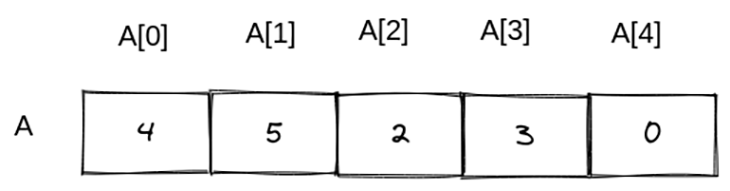Sometimes, we have to create multi-dimensional lists, such as for storing matrix details, we have to create a 2D list structure (a list inside a list). Moreover, for storing 3D rendering details, we need a 3D list structure.

We can store a simple matrix with the following 2D list structure.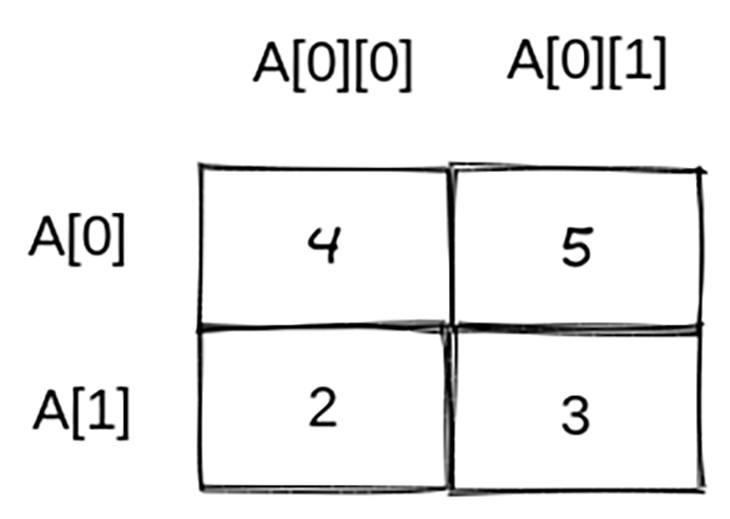It’s possible to convert the above diagram into the following Python code snippet.

```A = [[4, 5], [2, 3]]
print(A) #[[4, 5], [2, 3]]
```

Now, we need to use the square bracket-based index syntax twice to get a stored value. For example, you would need to write `A\[0\]` to get the value `5`.

Similarly, you can create N-D lists according to your requirements, such as 3D lists, 4D lists, etc.

## Mapping and filtering

Sometimes, we need to transform list elements into different data types. For example, we often have to convert string lists to integer lists. Moreover, sometimes we have to apply custom transformations. In those scenarios, we can use the inbuilt `map` function without using traditional loops.

Let’s convert a user-entered string list to an integers list with the following code.

```A = input('Enter some numbers: ').strip().split(' ')
A = list(map(int, A))
print(A)
```

When you run the above code, you will see the following result.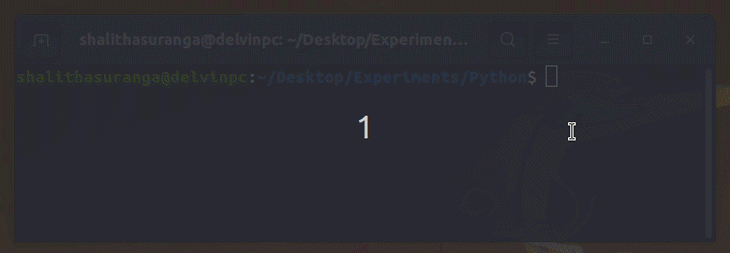Note that `int` is a function here  and not a data type. Therefore, we can write a custom function to transform data elements. Look at the following code that multiplies all of our list items by two.

```A = [1, 2, 3, 4]

def multiply_by_two(x):
return x * 2

B = list(map(multiply_by_two, A))
print(B) # [2, 4, 6, 8]
```

Filtering can also be used to remove specific items from a list based on a condition. For example, the following code removes all odd numbers.

```A = [1, 2, 3, 4, 5, 6, 7, 8]

def is_even(x):
return x % 2 == 0

A = list(filter(is_even, A))
print(A) # [2, 4, 6, 8]
```

The inbuilt `filter` function calls `is_even` for every item by sending each item to the `is_even` function. We need to return `True` if we need to include the given item. Therefore, `is_even` returns `True` if `x` is even.

## Conclusion

In this tutorial, we discussed Python lists with various practical examples. Python offers inbuilt methods for basic list manipulation requirements such as sorting, reversing, filtering, and mapping. These generic Python list solutions allow you to store a list of arbitrary elements, but if you would like to store only numbers for scientific computing with better performance, the NumPy package’s array structure is a great alternative.

It’s worth remembering that lists are not always suitable, and you’ll still need to learn other collection types as well. For example, if you need to create a read-only list, you can use the inbuilt tuple type. As lists allow duplicate data elements, you may instead want to use a Python set if you need to make a list with unique data elements without implementing additional logic by wrapping a list structure.

# Get set up with LogRocket's modern error tracking in minutes:

1. Visit https://logrocket.com/signup/ to get an app ID
2. Install LogRocket via npm or script tag. `LogRocket.init()` must be called client-side, not server-side
3. `\$ npm i --save logrocket // Code:import LogRocket from 'logrocket'; LogRocket.init('app/id');`
`Add to your HTML:<script src="https://cdn.lr-ingest.com/LogRocket.min.js"></script><script>window.LogRocket && window.LogRocket.init('app/id');</script>`
4. (Optional) Install plugins for deeper integrations with your stack:
• Redux middleware
• NgRx middleware
• Vuex pluginShalitha Suranga Programmer | Author of Neutralino.js | Technical Writer

## 2 Replies to “Working with lists in Python”

1.PyCode says:

Thanks For This Great tutorial about Python list!

2.Lee Frates says:

Very well discussed Python lists with various practical examples. Thanks for this tutorial.